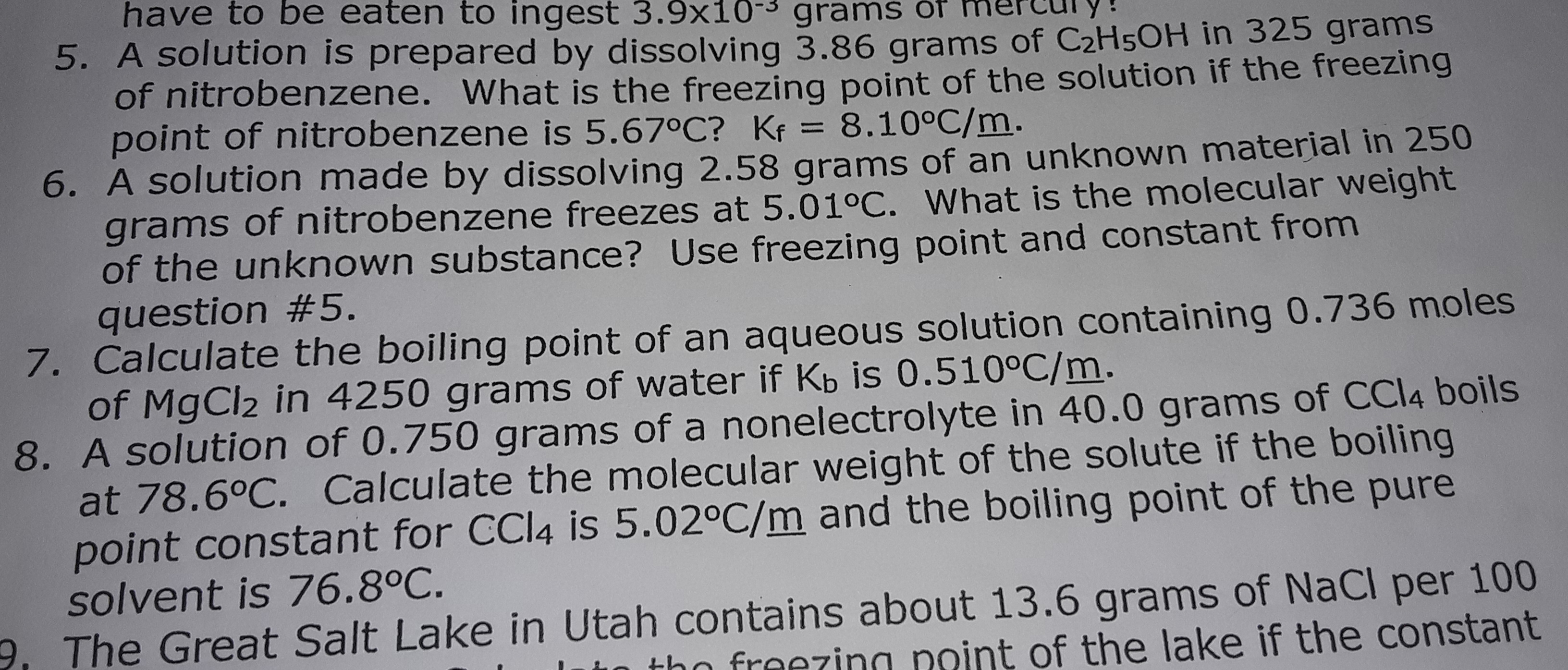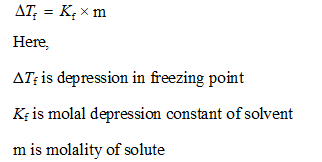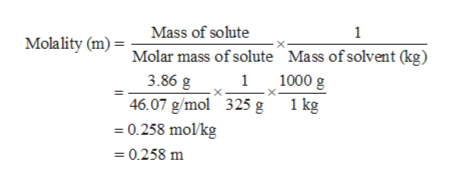# have to be eaten to ingest 3.9x10 gramS5. A solution is prepared by dissolving 3.86 grams of C2H5OH in 325 gramsof nitrobenzene. What is the freezing point of the solution if the freezingpoint of nitrobenzene is 5.67°C? Kf = 8.10°C/m.6. A solution made by dissolving 2.58 grams of an unknown material in 250grams of nitrobenzene freezes at 5.01°C. What is the molecular weightof the unknown substance? Use freezing point and constant fromquestion #5.7. Calculate the boiling point of an aqueous solution containing 0.736 molesof MgCl2 in 4250 grams of water if Kb is 0.510°C/m.8. A solution of 0.750 grams of a nonelectrolyte in 40.0 grams of CCI4 boilsat 78.6°C. Calculate the molecular weight of the solute if the boilingpoint constant for CCI4 is 5.02°C/m and the boiling point of the puresolvent is 76.8°C.The Great Salt Lake in Utah contains about 13.6 grams of NaCl per 100fraezinn noint of the lake if the constant

Question
9 views

Questions 5& 6help_outlineImage Transcriptionclosehave to be eaten to ingest 3.9x10 gramS 5. A solution is prepared by dissolving 3.86 grams of C2H5OH in 325 grams of nitrobenzene. What is the freezing point of the solution if the freezing point of nitrobenzene is 5.67°C? Kf = 8.10°C/m. 6. A solution made by dissolving 2.58 grams of an unknown material in 250 grams of nitrobenzene freezes at 5.01°C. What is the molecular weight of the unknown substance? Use freezing point and constant from question #5. 7. Calculate the boiling point of an aqueous solution containing 0.736 moles of MgCl2 in 4250 grams of water if Kb is 0.510°C/m. 8. A solution of 0.750 grams of a nonelectrolyte in 40.0 grams of CCI4 boils at 78.6°C. Calculate the molecular weight of the solute if the boiling point constant for CCI4 is 5.02°C/m and the boiling point of the pure solvent is 76.8°C. The Great Salt Lake in Utah contains about 13.6 grams of NaCl per 100 fraezinn noint of the lake if the constant fullscreen
check_circle

Step 1

Since,  we answer only one question at a time, we'll be providing the answer to question 5. Please submit question 6 separately.

Step 2

Depression in freezing point is a colligative property. It is the decrease in freezing point of solvent due to presence of solute (volatile or non-volatile) in it.

Its value depends on the number of moles of solute. It is related to molality by the expression:Step 3

Solute is ethanol and solvent is nitrobenzene.

Mass of ethanol = 3.86 g

Molar mass of ethanol = 46.07 g/mol...help_outlineImage TranscriptioncloseMass of solute Molality (m) = Molar mass of solute Mass ofsolvent (kg) 1 11000 g 3.86 g 46.07 g/mol 325 g 0.258 mol/kg 1 kg =0.258 m fullscreen

### Want to see the full answer?

See Solution

#### Want to see this answer and more?

Solutions are written by subject experts who are available 24/7. Questions are typically answered within 1 hour.*

See Solution
*Response times may vary by subject and question.
Tagged in

### Chemistry